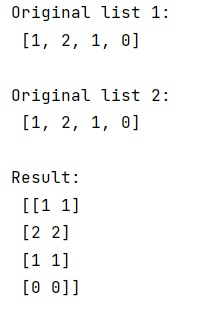# How to convert two lists into a matrix?

Given two Python lists, we have to convert them into a NumPy matrix.
Submitted by Pranit Sharma, on February 17, 2023

NumPy is an abbreviated form of Numerical Python. It is used for different types of scientific operations in python. Numpy is a vast library in python which is used for almost every kind of scientific or mathematical operation. It is itself an array which is a collection of various methods and functions for processing the arrays.

## Converting two lists into a matrix

Suppose that we are given two 1D lists and we need to convert these lists into a matrix.

For this purpose, we can use numpy.column_stack() which is used to stack 1-D arrays as columns into a 2-D array. It takes a sequence of 1-D arrays and stacks them as columns to make a single 2-D array. 2-D arrays are stacked as-is, just like with hstack.

1-D arrays are turned into 2-D columns first. It takes a single parameter called tup which is a sequence of 1-d or 2-D arrays and it returns an array formed by stacking the given arrays.

Let us understand with the help of an example,

## Python code to convert two lists into a matrix

```# Import numpy
import numpy as np

# Creating two lists
l1 = [1,2,1,0]
l2 = [1,2,1,0]

# Display original lists
print("Original list 1:\n",l1,"\n")
print("Original list 2:\n",l2,"\n")

# Converting two lists into a matrix
res = np.column_stack((l1,l2))

# Display Result
print("Result:\n",res)
```

Output: